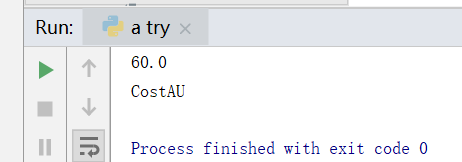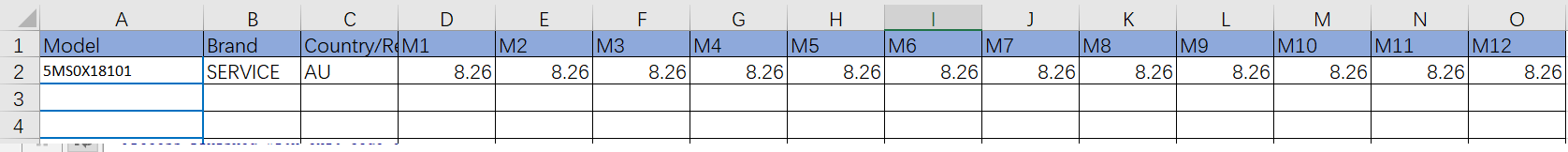``````import openpyxl
import xlrd

def get_infor():
book = xlrd.open_workbook('C:/Users/lenovo/Desktop/模板.xlsx')
sheet = book.sheet_by_name('WRT模板')
L =[]
for i in range (3,sheet.nrows):          # i 从第四列开始
PN = str(sheet.cell(i,3).value)
Cost_USD = str(sheet.cell(i, 7).value)
L.append((PN,Cost_USD))
#t=tuple(L)
return L
#print(L)

t1= get_infor()
#
# print(t1)
#
# sh = t1

# print(sh)
#
def Open_excel():
sheet = workbook.worksheets   # 第一个页签
sheet['D2'] = t1
#sheet['A1'] = t1
print(sheet['D2'].value)
print(sheet.title)

Open_excel()

``````2个回答

workbook.save('C:/Users/lenovo/Desktop/文档模板/AU/Cost_AU.xlsx')3 个月之前 回复James the Tomato� HI 朋友，这个我也怀疑过，然而貌似并不是这个原因 ：）
3 个月之前 回复